# Linux 直接I/O 原理与实现Linux内核那些事 |18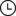4天前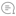0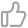00

## 缓存I/O

``int open(const char *pathname, int flags, ... /*, mode_t mode */ );``

• `pathname`：指定要打开的文件路径。

• `flags`：指定打开文件的标志。

• `mode`：可选，指定打开文件的权限。

O_RDONLY以只读的方式打开文件
O_WRONLY以只写的方式打开文件
O_RDWR以读写的方式打开文件
O_CREAT若文件不存在，则创建该文件
O_EXCL以独占模式打开文件；若同时设置 O_EXCL 和 O_CREATE, 那么若文件已经存在，则打开操作会失败
O_NOCTTY若设置该描述符，则该文件不可以被当成终端处理
O_TRUNC截断文件，若文件存在，则删除该文件
O_APPEND若设置了该描述符，则在写文件之前，文件指针会被设置到文件的底部
O_NONBLOCK以非阻塞的方式打开文件
O_NELAY同 O_NELAY，若同时设置 O_NELAY 和 O_NONBLOCK，O_NONBLOCK 优先起作用
FASYNC若设置该描述符，则 I/O 事件通知是通过信号发出的
O_SYNC该描述符会对普通文件的写操作产生影响，若设置了该描述符，则对该文件的写操作会等到数据被写到磁盘上才算结束
O_DIRECT该描述符提供对直接 I/O 的支持
O_LARGEFILE该描述符提供对超过 2GB 大文件的支持
O_DIRECTORY该描述符表明所打开的文件必须是目录，否则打开操作失败
O_NOFOLLOW若设置该描述符，则不解析路径名尾部的符号链接

`flags` 参数用于指定打开文件的标志，比如指定 `O_RDONLY`，那么就只能以只读方式对文件进行读写。这些标志都能通过 `位或 (|)` 操作来设置多个标志如：

``open("/path/to/file", O_RDONLY|O_APPEND|O_DIRECT);``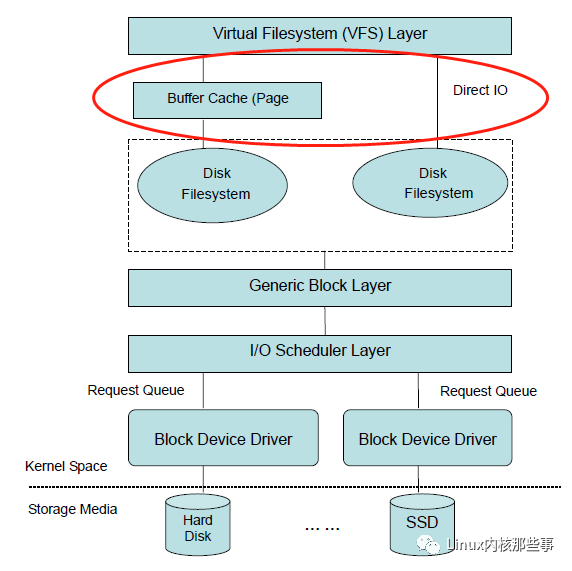## 缓存I/O 的优缺点

`缓存I/O` 的引入是为了减少对块设备的 I/O 操作，但是由于读写操作都先要经过缓存，然后再从缓存复制到用户空间，所以多了一次内存复制操作。如下图所示：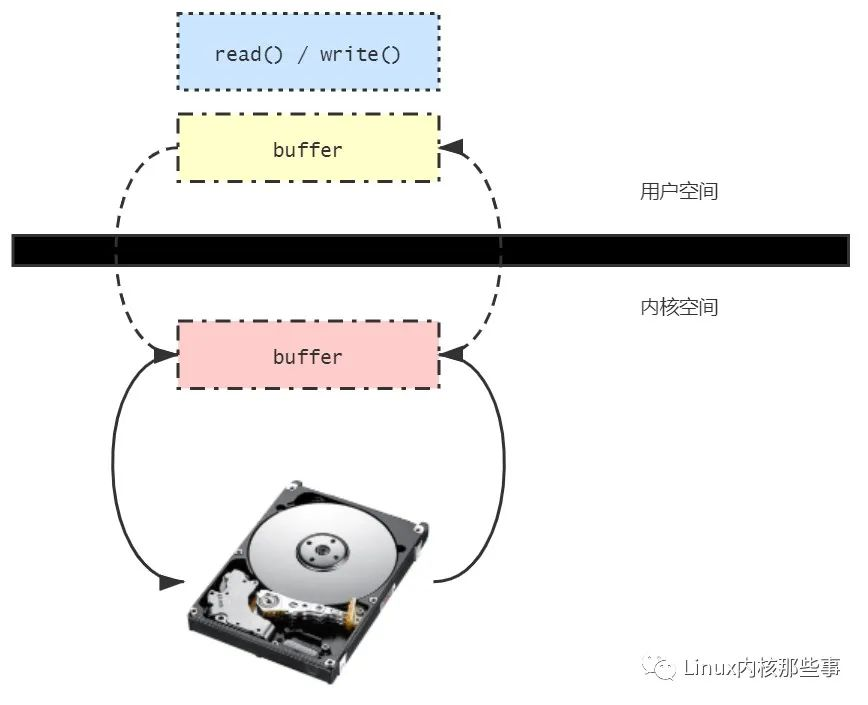## 直接I/O

`直接I/O` 就是对用户进行的 I/O 操作直接与块设备进行交互，而不进行缓存。

• `直接I/O` 的优点是：由于不对 I/O 数据块进行缓存，所以可以直接跟用户数据进行交互，减少一次内存的拷贝。

• `直接I/O` 的缺点是：每次 I/O 操作都直接与块设备进行交互，增加了对块设备的读写操作。

## 直接I/O 实现

``asmlinkage long sys_open(const char *filename, int flags, int mode)``{``    char *tmp;``    int fd, error;``    ...``    tmp = getname(filename); // 把文件名从用户空间拷贝到内核空间``    fd = PTR_ERR(tmp);``    if (!IS_ERR(tmp)) {``        fd = get_unused_fd(); // 申请一个还没有使用的文件描述符``        if (fd >= 0) {``            // 根据文件路径打开文件, 并获取文件对象``            struct file *f = filp_open(tmp, flags, mode);``            error = PTR_ERR(f);``            if (IS_ERR(f))``                goto out_error;``            fd_install(fd, f); // 把文件对象与文件描述符关联起来``        }``out:``        putname(tmp);``    }``    return fd;``    ...``}``

``struct file *dentry_open(struct dentry *dentry, struct vfsmount *mnt, int flags)``{``    struct file *f;``    ...``    f = get_empty_filp();``    f->f_flags = flags;``    ...``}``

``asmlinkage ssize_t sys_read(unsigned int fd, char *buf, size_t count)``{``    ssize_t ret;``    struct file *file;``    file = fget(fd);``    if (file) {``        ...``            if (!ret) {``                ssize_t (*read)(struct file *, char *, size_t, loff_t *);``                ret = -EINVAL;``                // ext2文件系统对应的是: generic_file_read() 函数``                if (file->f_op && (read = file->f_op->read) != NULL)``                    ret = read(file, buf, count, &file->f_pos);``            }``        ...``    }``    return ret;``}``

``ssize_t generic_file_read(struct file *filp, char * buf, size_t count, loff_t *ppos)``{``    ssize_t retval;``    ...``    if (filp->f_flags & O_DIRECT) // 如果标记了使用直接IO``        goto o_direct;``    ...`` o_direct:``    {``        loff_t pos = *ppos, size;``        struct address_space *mapping = filp->f_dentry->d_inode->i_mapping;``        struct inode *inode = mapping->host;``        ...``        size = inode->i_size;``        if (pos < size) {``            if (pos + count > size)``                count = size - pos;``            retval = generic_file_direct_IO(READ, filp, buf, count, pos);``            if (retval > 0)``                *ppos = pos + retval;``        }``        UPDATE_ATIME(filp->f_dentry->d_inode);``        goto out;``    }``}``

``static ssize_t generic_file_direct_IO(int rw, struct file *filp, char *buf, size_t count, loff_t offset)``{``    ...``    while (count > 0) {``        iosize = count;``        if (iosize > chunk_size)``            iosize = chunk_size;``        // 为用户虚拟内存空间申请物理内存页``        retval = map_user_kiobuf(rw, iobuf, (unsigned long)buf, iosize);``        if (retval)``            break;``        // ext2 文件系统对应 ext2_direct_IO() 函数,``        // 而 ext2_direct_IO() 函数直接调用了 generic_direct_IO() 函数``        retval = mapping->a_ops->direct_IO(rw, inode, iobuf, (offset+progress) >> blocksize_bits, blocksize);``        ...``    }``    ...``}``

`generic_file_direct_IO()` 函数主要的处理有两部分：

• 调用 `map_user_kiobuf()` 函数为用户虚拟内存空间申请物理内存页。

• 调用真实文件系统的 `direct_IO()` 接口对 `直接I/O` 进行处理。

`map_user_kiobuf()` 函数属于内存管理部分，可以参考之前的 内存管理 相关的文章进行分析，这里就不重复了。

`generic_file_direct_IO()` 函数最终会调用真实文件系统的 `direct_IO()` 接口，对于 `ext2文件系统``direct_IO()` 接口对应的是 `ext2_direct_IO()` 函数，而 `ext2_direct_IO()` 函数只是简单的封装了 `generic_direct_IO()` 函数，所以我们来分析下 `generic_direct_IO()` 函数的实现：

``int generic_direct_IO(int rw, struct inode *inode, struct kiobuf *iobuf,``        unsigned long blocknr, int blocksize, get_block_t *get_block)``{``    int i, nr_blocks, retval;``    unsigned long *blocks = iobuf->blocks;``    nr_blocks = iobuf->length / blocksize;``    // 获取要读取的数据块号列表``    for (i = 0; i < nr_blocks; i++, blocknr++) {``        struct buffer_head bh;``        bh.b_state = 0;``        bh.b_dev = inode->i_dev;``        bh.b_size = blocksize;``        retval = get_block(inode, blocknr, &bh, rw == READ ? 0 : 1);``        ...``        blocks[i] = bh.b_blocknr;``    }``    // 开始进行I/O操作``    retval = brw_kiovec(rw, 1, &iobuf, inode->i_dev, iobuf->blocks, blocksize);`` out:``    return retval;``}``

`generic_direct_IO()` 函数的逻辑也比较简单，首先调用 `get_block()` 获取要读取的数据块号列表，然后调用 `brw_kiovec()` 函数进行 I/O 操作。所以 `brw_kiovec()` 函数才是 I/O 操作的最终触发点。我们继续分析：

``int brw_kiovec(int rw, int nr, struct kiobuf *iovec[],``           kdev_t dev, unsigned long b[], int size)``{``    ...``    for (i = 0; i < nr; i++) {``        ...``        for (pageind = 0; pageind < iobuf->nr_pages; pageind++) {``            map  = iobuf->maplist[pageind];``            ...``            while (length > 0) {``                blocknr = b[bufind++];``                ...``                tmp = bhs[bhind++];``                tmp->b_size = size;``                set_bh_page(tmp, map, offset); // 设置保存I/O操作后的数据的内存地址 (用户空间的内存)``                tmp->b_this_page = tmp;``                init_buffer(tmp, end_buffer_io_kiobuf, iobuf); // 设置完成I/O后的收尾工作回调函数为: end_buffer_io_kiobuf()``                tmp->b_dev = dev;``                tmp->b_blocknr = blocknr;``                tmp->b_state = (1 << BH_Mapped) | (1 << BH_Lock) | (1 << BH_Req);``                ...``                submit_bh(rw, tmp); // 提交 I/O 操作 (通用块I/O层)``                if (bhind >= KIO_MAX_SECTORS) {``                    kiobuf_wait_for_io(iobuf);``                    err = wait_kio(rw, bhind, bhs, size);``                    ...``                }``            skip_block:``                length -= size;``                offset += size;``                if (offset >= PAGE_SIZE) {``                    offset = 0;``                    break;``                }``            } /* End of block loop */``        } /* End of page loop */``    } /* End of iovec loop */``    ...``    return err;``}``

`brw_kiovec()` 函数主要完成 3 个工作：

• 设置用于保存 I/O 操作后的数据的内存地址 (用户申请的内存)。

• 设置 I/O 操作完成后的收尾回调函数为: end_buffer_io_kiobuf()。

• 提交 I/O 操作到通用块层。00

暂无评论~~
Ctrl+Enter